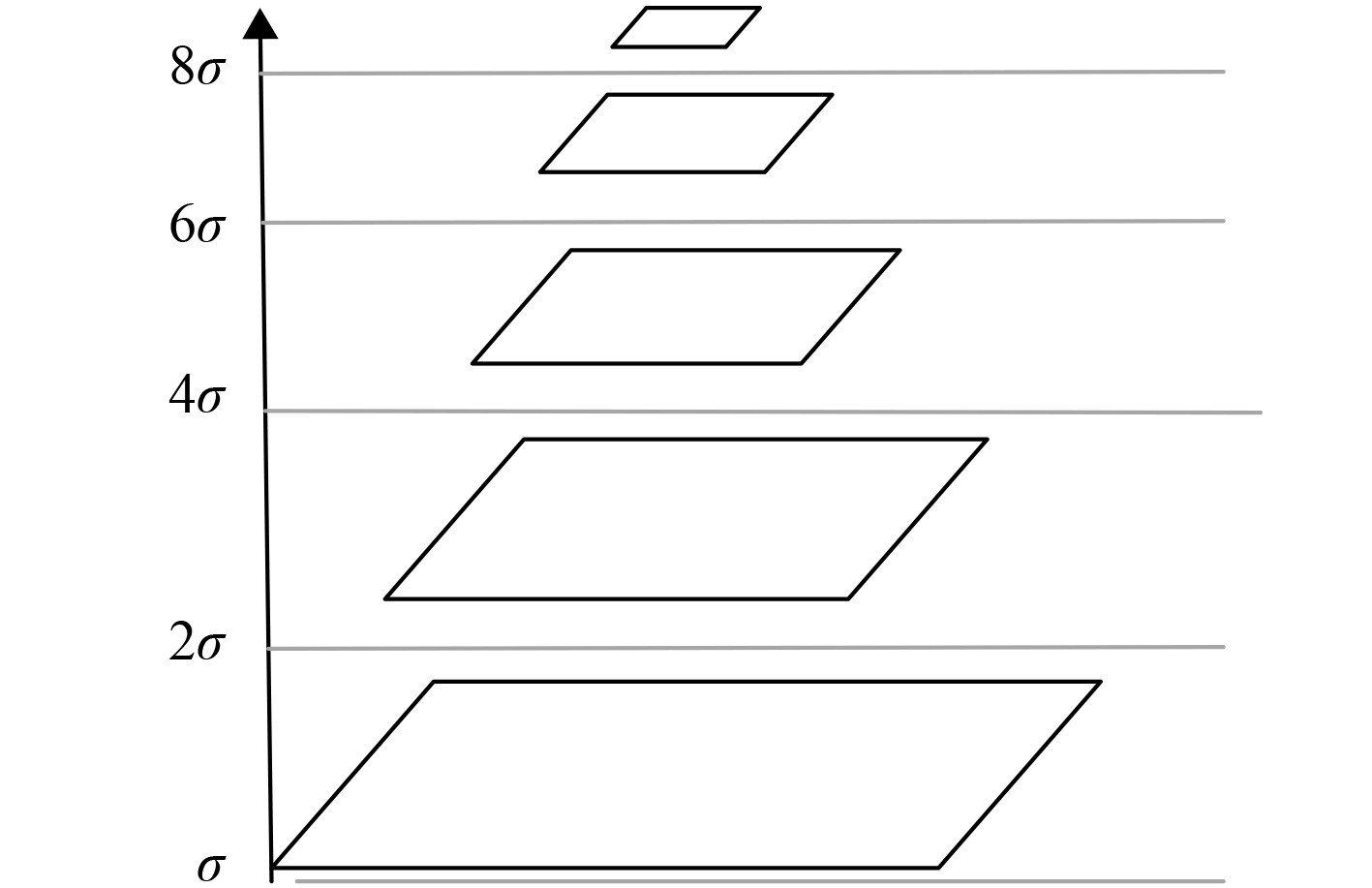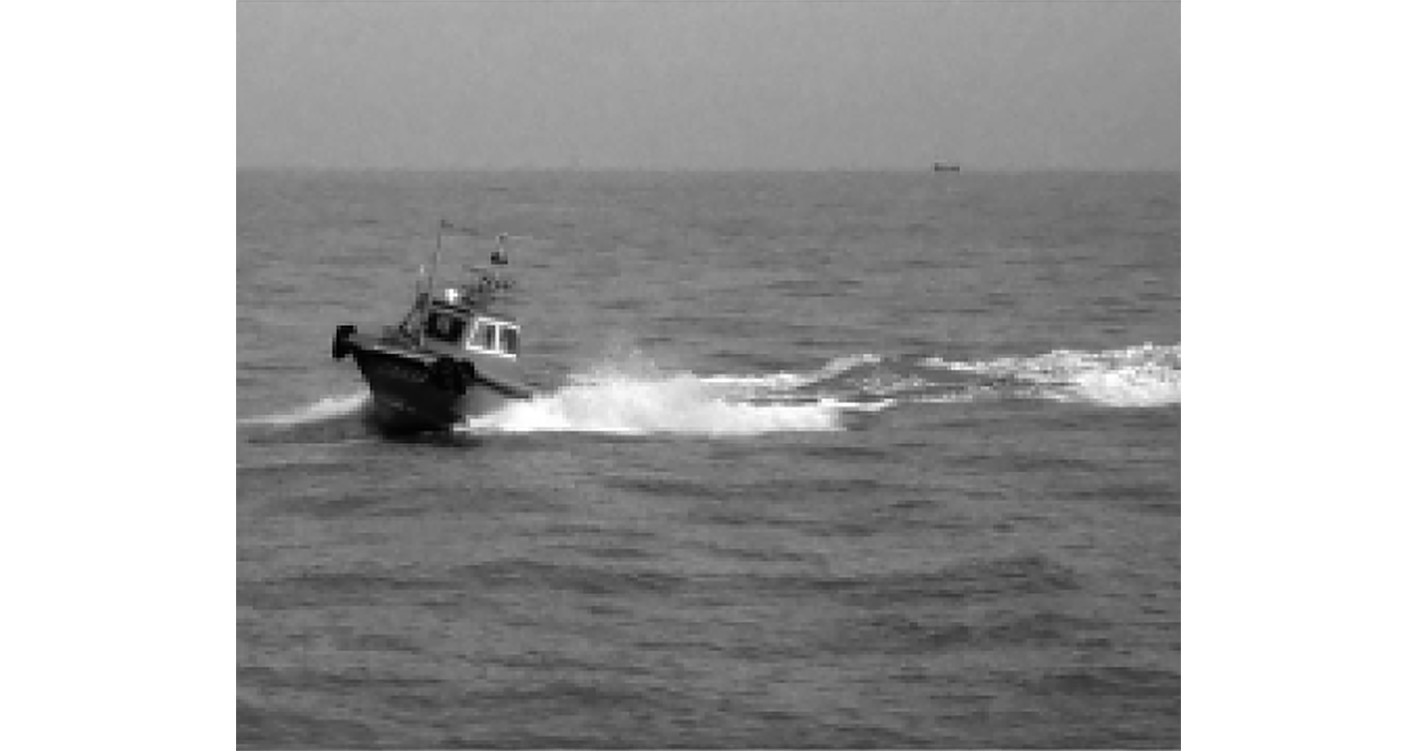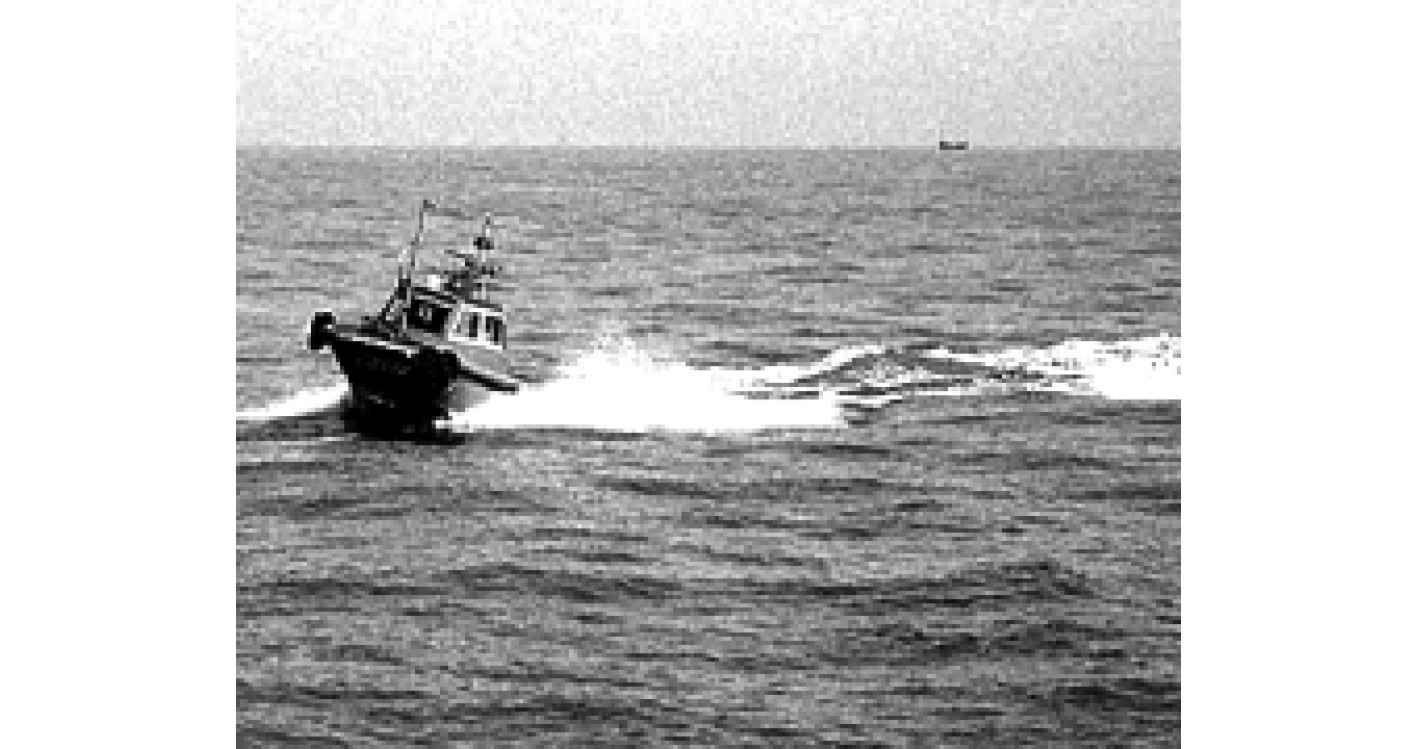﻿ 多尺度特征提取的复杂舰船图像分割研究
 舰船科学技术2022, Vol. 44Issue (7): 178-181    DOI: 10.3404/j.issn.1672-7649.2022.07.037PDF

1. 四川大学 电子信息学院，四川 成都 610065;
2. 四川城市职业学院 信息技术学院，四川 成都 610101;
3. 泸州职业技术学院 人工智能与大数据学院，四川 泸州 646000

Research on complex ship image segmentation based on multi-scale feature extraction
ZHOU Fan1,2, WANG Yang3
1. College of Electronics and Information Engineering, Sichuan University, Chengdu 610065, China;
2. Department of information technology, Urban Vocational College of Sichuan, Chengdu 610101, China;
3. School of Artificial Intelligence and Big Data, Luzhou Vocational and Technical College, Luzhou 646000, China
Abstract: The quality of image segmentation is directly related to the accuracy and computation of subsequent image recognition. In order to achieve high-quality and efficient segmentation of ship image, a complex ship image segmentation method based on multi-scale feature extraction is studied. This method carries out gray-scale and filtering preprocessing for complex ship images. Histogram equalization method is used to improve the image contrast and highlight the target features. The image is decomposed by Gaussian pyramid, and four features of each level of scale image are extracted. After normalization, the feature vector is obtained. The mean shift algorithm is used to realize over segmentation, and the graph theory method is used to realize re segmentation. The results show that compared with the threshold method, region method and edge method, the dice similarity coefficient is larger and the segmentation overlap error is smaller, indicating that the segmentation performance of the studied method is higher and more accurate and detailed image segmentation is realized.
Key words: multiscale features     complex ship image     pretreatment     image contrast enhancement     image segmentation method
0 引　言

1 基于多尺度特征提取的复杂舰船图像分割方法

1.1 复杂舰船图像预处理

1）图像灰度化

 $Y\left( {i,j} \right) = \frac{{\left[ {R\left( {i,j} \right) + G\left( {i,j} \right) + B\left( {i,j} \right)} \right]}}{3}。$ (1)

2）图像滤波

 $Y\left( {i,j} \right) = \frac{{\displaystyle\sum\limits_{\left( {i,j} \right) \in s}^M {y\left( {i,j} \right)} }}{M} 。$ (2)

1.2 图像对比度增强

 ${f_{{Q_i}}} = \frac{\sum\limits_{i = 0}^k {{\psi _k}} }}{\psi }$ (3)

 ${V_k}(x,y) = \frac{1}{\sum\limits_{k = 1}^{M \cdot N} {{{\left( {\frac{1}{{{{\sqrt {\left( {{D_k}(x,y) + B} \right)} }^2}}}} \right)}^A}} }} \times {\left( {\frac{1}{{{{\sqrt {\left( {{D_k}(x,y) + B} \right)} }^2}}}} \right)^A}$ (4)

 $F\left( {{{\boldsymbol{Z}}_{M \cdot N}}} \right) = \sum\limits_{k = 1}^{M \cdot N} {{V_k}} \cdot{\xi _k}\left( {{{\boldsymbol{Z}}_{M \cdot N}}} \right) 。$ (5)

1.3 复杂舰船图像的多尺度特征提取图 1 高斯金字塔分解图像过程 Fig. 1 Gaussian pyramid decomposition image process表 1 四种特征提取方法 Tab.1 Four feature extraction methods

1）灰度均值提取

 $f1 = \frac{{\displaystyle\sum\limits_{i,j = 0}^{{A_k} \cdot {B_k}} T (i,j)}}{{{A_k} \cdot {B_k}}} 。$ (6)

2）形状特征提取图像形状特征以面积、周长以及紧密度来描述。其中，面积可以直接通过统计目标区域中所包含的像素点数量来确定；周长可以通过目标区域对比度最大边界线上像素点之间的距离和来计算；紧密度根据面积和周长的比例来计算，公式如下：

 $P = \dfrac{{\sqrt {\dfrac{{4S}}{{\text{π}} }} }}{L} 。$ (7)

3）纹理特征提取

4）角点提取

1.4 复杂舰船图像分割图 2 复杂舰船图像过分割流程 Fig. 2 Over segmentation process of complex ship image

2 算例分析与测试

2.1 样本图像集图 3 舰船样本图像 Fig. 3 Ship sample image
2.2 相关参数设置表 2 基本参数表 Tab.2 Related parameters
2.3 图像对比度增强效果图 4 舰船图像对比度增强结果 Fig. 4 Contrast enhancement results of ship image
2.4 评价指标

1）Dice相似系数

 $D = \sqrt {\frac{{{{\left| {P \cap P'} \right|}^2}}}{{\left| P \right| + \left| {P'} \right|}}} 。$ (8)

2）分割重叠误差

 $H = 1 - \sqrt {\frac{{\left| {P \cap P'} \right|}}{{\left| {P \cup P'} \right|}}} 。$ (9)

2.5 分割性能分析表 3 方法分割性能指标 Tab.3 Performance index of method segmentation
3 结　语

  赵浩光, 王平, 董超, 等. 结合多尺度视觉显著性的舰船目标检测[J]. 光学精密工程, 2020, 28(6): 1395-1403.  宁芊, 胡诗雨, 雷印杰, 等. 基于多尺度特征和注意力机制的航空图像分割[J]. 控制理论与应用, 2020, 37(6): 1218-1224.  张田, 田勇, 王子, 等. 基于清晰度评价的自适应阈值图像分割法[J]. 东北大学学报(自然科学版), 2020, 41(9): 1231-1238. DOI:10.12068/j.issn.1005-3026.2020.09.003  黄和尧, 李英娜. 一种基于二维Otsu阈值法改进的区域生长红外图像分割法[J]. 电视技术, 2020, 44(11): 63-67.  薛丽霞, 孙伟, 汪荣贵, 等. 基于边缘概率的层次交互式图像分割算法[J]. 合肥工业大学学报(自然科学版), 2020, 43(3): 342-348. DOI:10.3969/j.issn.1003-5060.2020.03.010  左向梅, 武亮. 基于深度图像分割的场景物体识别与匹配[J]. 工程技术研究, 2019, 4(17): 219-221. DOI:10.3969/j.issn.1671-3818.2019.17.102  杨龙, 苏娟, 黄华, 等. 一种基于深层次多尺度特征融合CNN的SAR图像舰船目标检测算法[J]. 光学学报, 2020, 40(2): 132-140.  霍星, 张飞, 邵堃, 等. 改进的元启发式优化算法及其在图像分割中的应用[J]. 软件学报, 2021, 32(11): 3452-3467.  杨蕴, 李玉, 赵泉华. 基于局部空间信息的可变类模糊阈值光学遥感图像分割[J]. 自动化学报, 2022, 48(2): 582-593.  刘侠, 甘权, 刘晓, 等. 基于超像素的联合能量主动轮廓CT图像分割方法[J]. 光电工程, 2020, 47(1): 12-21.